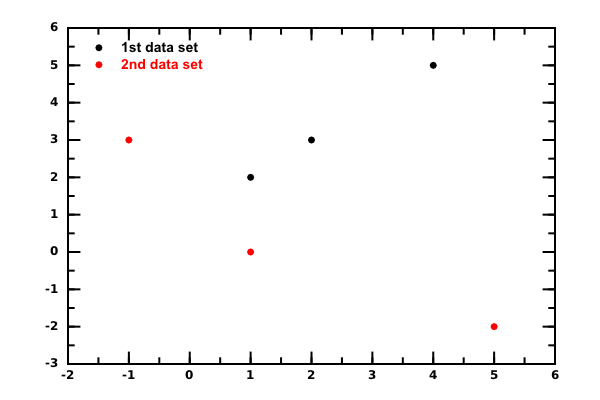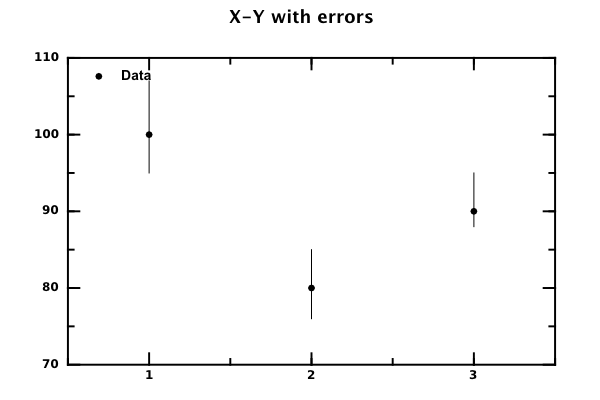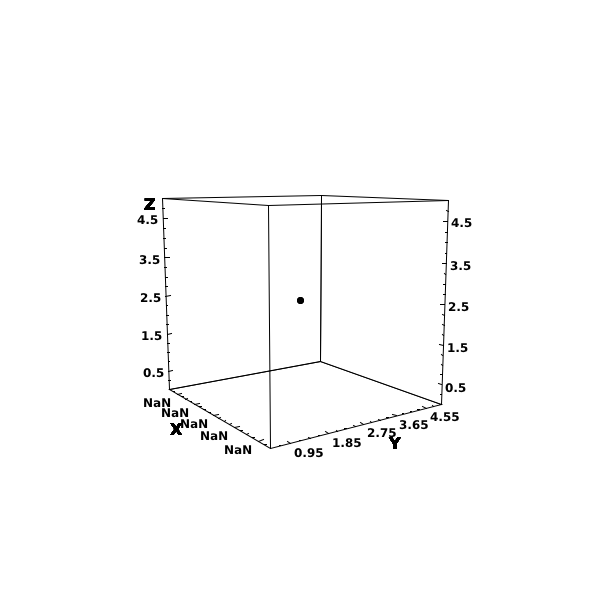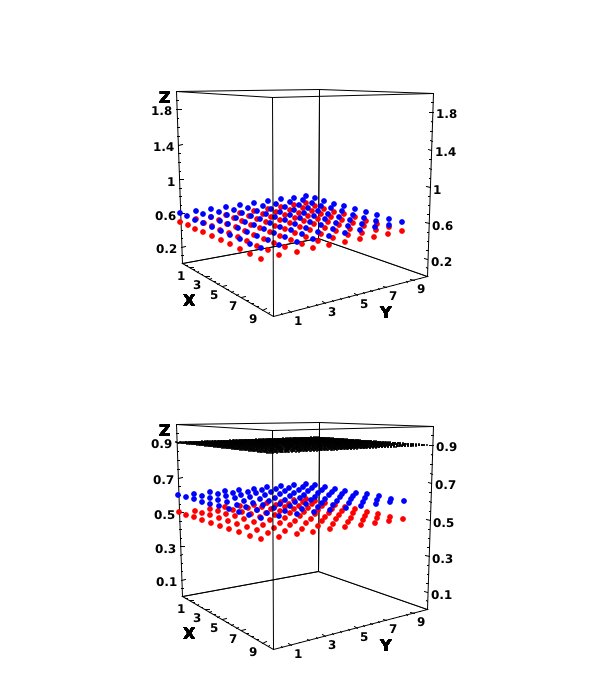# DMelt:DataAnalysis/1 Data Structures

Limitted access. First login to DataMelt if you are a full DataMelt member. Then login to HandWiki as a user.

# Data structures

The DataMelt data containers are designed for scientific data analysis and are well suited for data manipulation, input/output and data representation using various canvases. Most popular examples are:

# 1D-arrays. P0D and P0I classes

Such arrays are based on the Java classjhplot.P0D. One can fill such arrays using the method "add" and display its content. The statistical summary can also be easily obtained:

The example below shows how to build such arrays using the Python syntax. We fill an array with 10 sequential numbers from 0 to 9 and then we convert it into a string (for printing). Finally, we evaluate a complete statistical summary using the "getStat" method:

from jhplot  import  *
p0=P0D("test")
for i in range(10):
print p0.toString()

This prints in the prompt:

P0D test
0.0
1.0
2.0
3.0
4.0
5.0
6.0
7.0
8.0
9.0

One can view the data containers using several methods. One is "toString()" which converts data into a string. One can write data into a file (including a compression) using the method "toFile(file)"). One can also view data in a sortable table using the method "toTable()", or calling the "HTable(obj)" directly, where "obj" is one of the containers discusses above. This works even for DataMelt histograms. For example, the executing this line after the above example is

HTable(p0)

brings up a table where data can be sorted and searched.

As for any DataMelt data object, one can write and read arrays into files using the method "toFile" and read using the method "read()"

p0.toFile("data.txt")

p0.read("data.txt")

If a file was zipped use the method "readZip()". One can access various statistical characteristics of thejhplot.P0D arrays as:

print p0.getStatString()

The output of this script is shown below:

cern.hep.aida.bin.DynamicBin1D
-------------
Size: 10
Sum: 45.0
SumOfSquares: 285.0
Min: 0.0
Max: 9.0
Mean: 4.5
RMS: 5.338539126015656
Variance: 9.166666666666666
Standard deviation: 3.0276503540974917
Standard error: 0.9574271077563381
Geometric mean: 0.0
Product: 0.0
Harmonic mean: 0.0
Sum of inversions: Infinity
Skew: 0.0
Kurtosis: -1.5616363636363637
Sum of powers(3): 2025.0
Sum of powers(4): 15333.0
Sum of powers(5): 120825.0
Sum of powers(6): 978405.0
Moment(0,0): 1.0
Moment(1,0): 4.5
Moment(2,0): 28.5
Moment(3,0): 202.5
Moment(4,0): 1533.3
Moment(5,0): 12082.5
Moment(6,0): 97840.5
Moment(0,mean()): 1.0
Moment(1,mean()): 0.0
Moment(2,mean()): 8.25
Moment(3,mean()): 0.0
Moment(4,mean()): 120.8625
Moment(5,mean()): 0.0
Moment(6,mean()): 2079.515625
25%, 50%, 75% Quantiles: 2.25, 4.5, 6.75
quantileInverse(median): 0.55
Distinct elements: [0.0, 1.0, 2.0, 3.0, 4.0, 5.0, 6.0, 7.0, 8.0, 9.0]
Frequencies: [1, 1, 1, 1, 1, 1, 1, 1, 1, 1]

You can access all such characteristics using the method getStat() which return Java Map (or Jython dictionary) where the key identifies each statistical value.

Since it is useful to re-bin 1D arrays using Histograms, consider usingjhplot.HPlotJas canvas which offers a GUI with a slider for on-fly rebinning. This is explained in more detail in the Section Interactive fit.

# 2D arrays. P1D class

2D arrays are based on the Java classjhplot.P1D. This is one of the reachest data collections that can be used to keep $(X,Y)$ values, (X,Y, Err) values, where "Err" is an error on "Y", and any (X,Y) values with asymmetric errors on X, Y.

As before, one can fill such arrays using the method "add(x,y)". It is one of the most advanced containers since data can contain up to 8 errors: 1st level (usually statistical) and 2nd level (usually systematic uncertainty). The dimension of this array can grow and shrink. If you need to keep only 2 values, X and Y, set the dimension of this object to 2 (which is anyway the default). But the dimension can also be up to 10, with 8 additional values necessary to set errors on X and Y.

This is also a high-performance container, which is faster than Jython list or java list. For example, you will need 2 Python list to keep X and Y. Instead, use a singlejhplot.P1D. Read about the performance of this container in Section man:data:data_collections.

The dimensions of this array are below. Use the "setDimension(dimension)" to initialize the container:

data dimension
X,Y 2 (default)
X,Y, errY-, errY+ (symmetric) 3
X,Y, errY-, errY+ 4
X,Y,errX-, errX+, errY-, errY+ 6
X,Y,errX-, errX+, errY-, errY+ + errXsys-, errXsys+, errYsys-, errYsys+ 10

Here are errY means a 1st level error (usually statistical), while errYsys means second-level errors (usually systematic).

For example, let us make a simple (X,Y) array:

from jhplot import *
p=P1D("XY")
p.add(x,y)

You can get arrays back as:

x=p.getArrayX()
y=p.getArrayY()

The main advantage in usingjhplot.P1D in its ability to handle various operations together with error propagation.

The example below shows how to build such arrays using the Python syntax. We fill an array with 10 sequential numbers from 0 to 9 and then we convert it into a string (for printing). Finally, we evaluate a complete statistical summary using the "getStat" method:

from jhplot  import  *
from java.awt import Color

p1=P1D("1st data set")

p2=P1D("2nd data set")
p2.setColor(Color.red)

c1 = HPlot("Canvas")
c1.visible()
c1.setAutoRange()

c1.draw(p1)
c1.draw(p2)

The output of this script is shown hereThejhplot.P1D data container can also show errors in X and Y for each data points, as well it has advanced mathematical operations with proper error propagation. For example,

p1.add(x,y,err) # fills X,Y and symmetric error on Y

where "err" is a statistical error on the Y value, assuming that yUpper=yLower. You can get values back as arrays using:

x=p1.getArrayX()
y=p1.getArrayX()
error=p1.getArrayErr()

If the error on Y is asymmetric, use this method:

p1.add(x,y,err_up, err_down)

where "err" and "err_down" are symmetric upper and lower error on Y.

The DataMelt contains advanced error propagation algorithms and can handle statistical errors (on X and Y) as well as systematic error (2nd level errors) Here is a small example which illustrate how to draw points with 1st-level (statistical) errors:

In this code, errors on the y-axis are asymmetric (jut to show that this is possible). The "add()" method has many variations, so one can assign errors for x-axis, y-axis (plus 2-level errors).# 3D- arrays. P2D class

Analogously, one can plot data in 3D. Usejhplot.P2D class to add values and plot them.from java.awt import Color
from jhplot  import HPlot3D,P2D

d1 = P2D("data1")
d2 = P2D("data2")
d3 = P2D("data3")
d1.setSymbolColor(Color.red)
d2.setSymbolColor(Color.blue)
d3.setSymbolSize(2)
for i in range(0,10):
for j in range(0,10):

for i in range(0,50):
for j in range(0,50):

c1 = HPlot3D("plot",600,700,1,2);
c1.setRange(0.0,10,0.0,10,0,2)
c1.visible()

c1.cd(1,1)
c1.draw(d1)
c1.draw(d2)

c1.cd(1,2)
c1.setRange(0.0,10,0.0,10,0.2,1.0)
c1.draw(d1)
c1.draw(d2)
c1.draw(d3)

Which generates the output:# Multi-dimensional arrays

Let us assume that we have a matrix of numbers organized as

# this is a multi-dimensional data
1 2 3 4
5 6 7 8
.......

(the numbers of rows and columns can be arbitrary). We can load and work with this data using thejhplot.PND class. A first step is to read the data into a DataMelt data container designed to keep such data and do some manipulation. Our preference is to read a data from a prepared file located on the Web:

<file python> from jhplot import * pn=PND('data','/dmelt/examples/data/pnd.d') print pn.toString() </jcode>

Here we create a PND object from the file "pnd.d" stored on the Web and print it for checking. The file has exactly the same structure as shown before, i.e. each row is separated by a new line. From now on, we use the Python syntax to print a string returned by the method "toString()". Alternatively, one can use "pn.toTable()" method to display all numbers in a sortable and searchable table. You will see the numbers printed out in the Jython shell (which is used for output of the print command).

Let us continue with the analysis of our data. First thing we want to do is to extract the numbers from the 2nd column and display Assuming that the "pn" object is created as shown before, we will extract the second column using the index 1 (the first column has the index 0)

p0=pn.getP0D(1)     # extract 2nd column and put to a 1D array
print p0.getStat()  # print a detailed statistical characteristics
c1=HPlot('Plot')    # create a canvas to display a histogram
c1.visible(); c1.setAutoRange()   # set auto-range
h1=p0.getH1D(10)    # convert 1D array into a histogram with 10 bins
c1.draw(h1)         # draw the histogram

The next step in our analysis is to extract the 2 columns and to make a X-Y scatter plot in order find a correlation between the numbers from these columns. In the example below we extract the 2nd and 3rd column, plot them on X-Y canvas and then perform a least-squared linear regression:

from jhplot.stat import *
p1=pn.getP1D(1,2)      # extract 2nd and 3rd columns
c1=HPlot('X-Y plot')
c1.visible(); c1.setAutoRange()  # set auto-range
c1.draw(p1)

This code should follow after the code which creates the object "pn" as discussed before. The execution of this code makes a X-Y graph with the values of the 2nd and 3rd columns